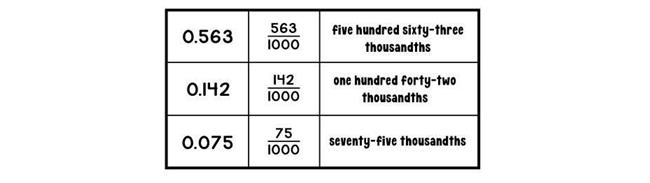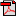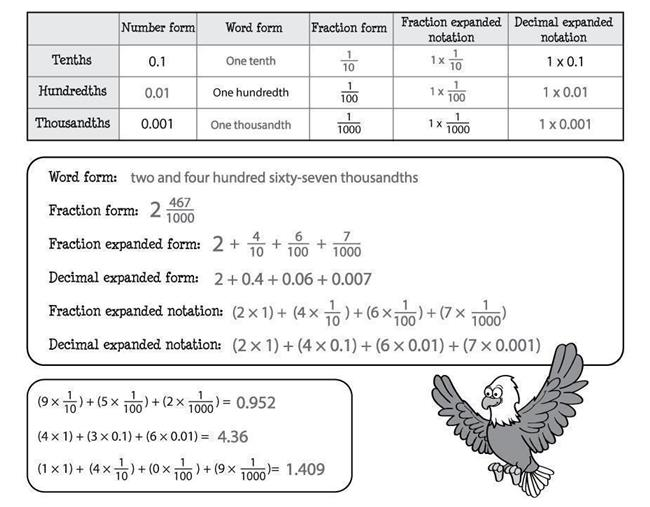# Lesson 4: Decimals to Thousandths

## Activities

### Activity 1: Getting to the Thousandths

Materials: fine point dry-erase markers (kit), laminated decimal grid (kit)
Look at the laminated decimal grid from your math kit. Each of the four grids represents a whole or the number 1. You should see that the Tenths grid is divided into 10 equal spaces. The Hundredths grid is divided into 100 equal spaces (10×10). So, how many equal spaces is the Thousandths grid divided into? You can check this mathematically by multiplying 100 times 10 because there are 100 small squares, and each of these squares is divided into 10 tiny columns: 100×10=1,000.
Use a dry-erase marker to show 0.6 on the Tenths grid and 0.06 on the Hundredths grid. You should color six spaces on each grid like this:Notice that 0.6 is much greater than 0.06. In the same way that digits in whole numbers grow larger in value as you move left, digits in decimal numbers grow smaller in value as you move right. The place to the right of the hundredths place is called the thousandths place. Here's what 0.006 or six thousandths looks like on the Thousandths grid.Here are examples of decimals to the thousandths and how you write and say them:Now, complete the "Thousandths" sheet by following the instructions provided. Use the laminated decimal grid as needed.Student Activity Page
During this activity, your child will use the laminated decimal grid sheet to identify decimal amounts to thousandths. Make sure that she has access to dry-erase markers.### Activity 2: Expanding Decimals

Materials: Interactive Notebook
You already know about writing whole numbers in expanded form and expanded notation. For example, 463 can be expanded to 400 + 60 + 3, and it can be expanded further into (4 × 100) + (6 × 10) + (3 × 1).

You can also write decimal numbers in both expanded form and expanded notation. Both expanded form and expanded notation can be shown with fractions or decimals. Look at the examples of each below. The first one shows fraction expanded form.Here is the same number written in decimal expanded form:In fraction and decimal expanded form, you can see that you simply expand, or stretch out, the number so that each digit value is shown separately. Expanded notation works just a bit differently. Look at the example number written in fraction expanded notation:Expanded notation expands the number but does so by naming the digit and its place value. To see how this approach works, it's important to remember how to multiply whole numbers by unit fractions (fractions that have a numerator of 1). You multiply the numerator in the fraction (1) by the whole number and leave the denominator the same. So, (8 × 1/10) is the same as 8/10, and (2 × 1/100) is the same as 2/100. Did you notice that the resulting fractions are the same ones shown in fraction expanded form? That's how you can see that each of these methods are simply different ways to write the same number.
You can also write in expanded notation using decimals:Notice that the decimal after each digit tells the place value where the digit belongs. So, in this example, 8 belongs in the tenths place and 2 belongs in the hundredths place.

You may wonder why the zero in the ones place isn't included in expanded notation. When a number has only decimal values, a zero is often included in the ones place. Although it isn't required, it can help make the number easier to read. Since the 0 is not holding a set place between valued numbers (as with the 0 in 206, for example), it isn't included in expanded notation.

Now, you'll complete the "Expanding Decimals" sheets. Be sure to look closely at the examples provided. When you are finished, you can store the first page in your Interactive Notebook.Student Activity PageStudent Activity Page
Your child will extend her understanding of writing numbers in expanded form and expanded notation to writing decimals in both fraction and decimal expanded form and fraction and decimal expanded notation. To complete this activity, your child will review how to multiply whole numbers and unit fractions by multiplying the numerator by the whole number and leaving the denominator the same. If your child would benefit from further practice with this skill before moving forward, the following video may be useful.